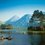# Please solve the math question

$Please\quad tell\quad me\quad the\quad solution\quad of\quad the\quad \\ following\quad question:\\ \\ In\quad \triangle ABC,\quad D\quad is\quad the\quad midpoint\quad of\quad \\ side\quad BC.\quad \angle ACB\quad =\quad { 30 }^{ \circ }.\quad \angle ADB\quad =\quad 45\\ Find\quad \angle BAD.\quad$ I know how to solve it using trigo. But tell me a different method. P.S it's an eighth class question.Note by Rajdeep Dhingra
6 years, 6 months ago

This discussion board is a place to discuss our Daily Challenges and the math and science related to those challenges. Explanations are more than just a solution — they should explain the steps and thinking strategies that you used to obtain the solution. Comments should further the discussion of math and science.

When posting on Brilliant:

• Use the emojis to react to an explanation, whether you're congratulating a job well done , or just really confused .
• Ask specific questions about the challenge or the steps in somebody's explanation. Well-posed questions can add a lot to the discussion, but posting "I don't understand!" doesn't help anyone.
• Try to contribute something new to the discussion, whether it is an extension, generalization or other idea related to the challenge.

MarkdownAppears as
*italics* or _italics_ italics
**bold** or __bold__ bold
- bulleted- list
• bulleted
• list
1. numbered2. list
1. numbered
2. list
Note: you must add a full line of space before and after lists for them to show up correctly
paragraph 1paragraph 2

paragraph 1

paragraph 2

[example link](https://brilliant.org)example link
> This is a quote
This is a quote
    # I indented these lines
# 4 spaces, and now they show
# up as a code block.

print "hello world"
# I indented these lines
# 4 spaces, and now they show
# up as a code block.

print "hello world"
MathAppears as
Remember to wrap math in $$ ... $$ or $ ... $ to ensure proper formatting.
2 \times 3 $2 \times 3$
2^{34} $2^{34}$
a_{i-1} $a_{i-1}$
\frac{2}{3} $\frac{2}{3}$
\sqrt{2} $\sqrt{2}$
\sum_{i=1}^3 $\sum_{i=1}^3$
\sin \theta $\sin \theta$
\boxed{123} $\boxed{123}$

Sort by:

DO YOU WANT FULL SOLUTION OR JUST HINT , IF HINT , THEN TRY BY CONSTRUCTION.

- 6 years, 6 months ago

- 6 years, 6 months ago

No,it's 30.

- 6 years, 6 months ago

- 6 years, 6 months ago

FULL SOLUTION

- 6 years, 6 months ago

- 6 years, 6 months ago

- 6 years, 6 months ago

I believe that this is an NMTC problem. You can view the solution discusssion in this problem. One approach just uses sine rule, while another approach gets more creative.

Staff - 6 years, 6 months ago